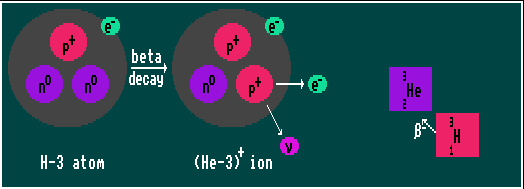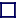### BETA DECAY

BETA DECAY (ELECTRON EMISSION)
A free neutron is unstable, decaying (with a half-life of about 11 minutes) by beta decay via the weak force into a proton, an electron and a neutrino. However, inside the nucleus of a stable nuclide, the neutron is stable. But if the nuclide has an excess of neutrons, it is again subject to beta decay. This only occurs if the atomic mass of the product nuclide is less than that of the source nuclide. After decay, the Atomic Number, Z, increases by one (the parent element transmutes into one of higher Atomic Number); but that the Atomic Weight (Z+N) has not changed. (e.g. Cl-40 becomes Ar-40).

Beta decay is usually accompanied by incidental gamma ray emission due to recoil energy.

Unlike in alpha decay, where alpha particles are emitted with specific energies, in BETA decay the electrons are emitted with a continuous range of energies because the momentum is divided randomly between two escaping particles, the electron and the neutrino (the proton stays within the nucleus). Superimposed on this continuous beta ray spectrum are several spectral lines, caused by the emitted GAMMA rays knocking extra electrons out from the inner orbit of the same atom. This is known as internal conversion.

Beta decay particle energies range from a few thousand electron volts to about 17 MeV, a much wider range of energies than in alpha decay. Halflives vary from about 10ms to 100 Giga years and are inversely proportional to the fifth power of the decay energy. In beta decay, the negatively charged electron emitted from the nucleus is attracted by the positively charged protons within the nucleus, and thus both the probability of decay and the particle energies are decreased. (The converse is true for inverse beta decay). Also, as proton number (Z) increases, so does the magnitude of this effect. The emerging relativistic electron is so energetic that it is barely affected by the surrounding and diffuse electron cloud.

Because beta decay involves the W- weak-force carrying particle and the weak force violates parity conservation, beta decay always produces left-handed electrons and right-handed anti-neutrinos, never the other way around. Conversely, inverse beta decay involves the W+ particle, and always produces right-handed positrons and left-handed neutrinos.

Shown is hydrogen-3 decaying into the stable helium-3 by beta decay, where an electron is liberated.